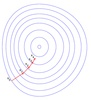• 详细来讲讲梯度下降算法的原理，感受数学和程序的魅力吧！！
博文目录1. 概述2. 梯度下降算法2.1 场景假设2.2 梯度下降2.2.1 微分2.2.2 梯度2.3 数学解释2.3.1 α2.3.2 梯度要乘以一个负号3. 实例3.1 单变量函数的梯度下降3.2 多变量函数的梯度下降4. 代码实现4. 1 场景分析4. 2 代码5. 小结
1. 概述
本文将从一个下山的场景开始，先提出梯度下降算法的基本思想，进而从数学上解释梯度下降算法的原理，解释为什么要用梯度，最后实现一个简单的梯度下降算法的实例！
2. 梯度下降算法
2.1 场景假设
梯度下降法的基本思想可以类比为一个下山的过程。
假设这样一个场景：一个人被困在山上，需要从山上下来(找到山的最低点，也就是山谷)。但此时山上的浓雾很大，导致可视度很低；因此，下山的路径就无法确定，必须利用自己周围的信息一步一步地找到下山的路。这个时候，便可利用梯度下降算法来帮助自己下山。怎么做呢，首先以他当前的所处的位置为基准，寻找这个位置最陡峭的地方，然后朝着下降方向走一步，然后又继续以当前位置为基准，再找最陡峭的地方，再走直到最后到达最低处；同理上山也是如此，只是这时候就变成梯度上升算法了2.2 梯度下降
梯度下降的基本过程就和下山的场景很类似。
首先，我们有一个可微分的函数。这个函数就代表着一座山。我们的目标就是找到这个函数的最小值，也就是山底。根据之前的场景假设，最快的下山的方式就是找到当前位置最陡峭的方向，然后沿着此方向向下走，对应到函数中，就是找到给定点的梯度 ，然后朝着梯度相反的方向，就能让函数值下降的最快！因为梯度的方向就是函数之变化最快的方向(在后面会详细解释)
所以，我们重复利用这个方法，反复求取梯度，最后就能到达局部的最小值，这就类似于我们下山的过程。而求取梯度就确定了最陡峭的方向，也就是场景中测量方向的手段。那么为什么梯度的方向就是最陡峭的方向呢？接下来，我们从微分开始讲起：
2.2.1 微分
看待微分的意义，可以有不同的角度，最常用的两种是：

函数图像中，某点的切线的斜率
函数的变化率
几个微分的例子：

1.单变量的微分，函数只有一个变量时
$\frac{d(x^2)}{dx}=2x$
$\frac{d(-2y^5)}{dy}=-10y^4$
$\frac{d(5-\theta )^2}{d\theta}=-2(5-\theta)$
2.多变量的微分，当函数有多个变量的时候，即分别对每个变量进行求微分
$\frac{\partial}{\partial x}(x^2y^2) = 2xy^2$
$\frac{\partial}{\partial y}(-2y^5+z^2) = -10y^4$
$\frac{\partial}{\partial \theta_{2}}(5\theta_{1} + 2\theta_{2} - 12\theta_{3}) = 2$
$\frac{\partial}{\partial \theta_{2}}(0.55 - (5\theta_{1} + 2\theta_{2} - 12\theta_{3})) = -2$
2.2.2 梯度
梯度实际上就是多变量微分的一般化。
下面这个例子：
$J(\Theta ) = 0.55 - (5\theta_{1} + 2\theta_{2} - 12\theta_{3})$
$\triangledown J(\Theta ) = \left < \frac{\partial J}{\partial \theta_{1}}, \frac{\partial J}{\partial \theta_{2}},\frac{\partial J}{\partial \theta_{3}} \right > =(-5,-2,12)$
我们可以看到，梯度就是分别对每个变量进行微分，然后用逗号分割开，梯度是用<>包括起来，说明梯度其实一个向量。
梯度是微积分中一个很重要的概念，之前提到过梯度的意义

在单变量的函数中，梯度其实就是函数的微分，代表着函数在某个给定点的切线的斜率
在多变量函数中，梯度是一个向量，向量有方向，梯度的方向就指出了函数在给定点的上升最快的方向

这也就说明了为什么我们需要千方百计的求取梯度！我们需要到达山底，就需要在每一步观测到此时最陡峭的地方，梯度就恰巧告诉了我们这个方向。梯度的方向是函数在给定点上升最快的方向，那么梯度的反方向就是函数在给定点下降最快的方向，这正是我们所需要的。所以我们只要沿着梯度的方向一直走，就能走到局部的最低点！
2.3 数学解释
首先给出数学公式：
${\color{Red} \Theta^1} = {\color{Blue} \Theta^0} + {\color{Green} \alpha} {\color{Purple} \triangledown J(\Theta)}\rightarrow evaluated at \Theta^0$
此公式的意义是：J是关于Θ的一个函数，我们当前所处的位置为Θ0点，要从这个点走到J的最小值点，也就是山底。首先我们先确定前进的方向，也就是梯度的反向，然后走一段距离的步长，也就是α，走完这个段步长，就到达了Θ1这个点！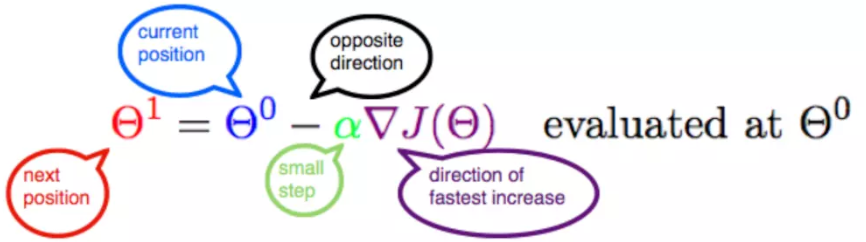2.3.1 α
α在梯度下降算法中被称作为学习率或者步长，意味着我们可以通过α来控制每一步走的距离，以保证不要步子跨的太大扯着蛋，哈哈，其实就是不要走太快，错过了最低点。同时也要保证不要走的太慢，导致太阳下山了，还没有走到山下。所以α的选择在梯度下降法中往往是很重要的！α不能太大也不能太小，太小的话，可能导致迟迟走不到最低点，太大的话，会导致错过最低点！
2.3.2 梯度要乘以一个负号
梯度前加一个负号，就意味着朝着梯度相反的方向前进！我们在前文提到，梯度的方向实际就是函数在此点上升最快的方向！而我们需要朝着下降最快的方向走，自然就是负的梯度的方向，所以此处需要加上负号；那么如果时上坡，也就是梯度上升算法，当然就不需要添加负号了。
3. 实例
我们已经基本了解了梯度下降算法的计算过程，那么我们就来看几个梯度下降算法的小实例，首先从单变量的函数开始，然后介绍多变量的函数。
3.1 单变量函数的梯度下降
我们假设有一个单变量的函数
$J(\theta) = \theta^2$
函数的微分，直接求导就可以得到
$J'(\theta) = 2\theta$
初始化，也就是起点，起点可以随意的设置，这里设置为1
$\theta^0 = 1$
学习率也可以随意的设置，这里设置为0.4
$\alpha = 0.4$
根据梯度下降的计算公式
${\color{Red} \Theta^1} = {\color{Blue} \Theta^0} + {\color{Green} \alpha} {\color{Purple} \triangledown J(\Theta)}\rightarrow evaluated at \Theta^0$
我们开始进行梯度下降的迭代计算过程：
$\theta^0 = 1$
$\theta^1 = \theta^0 - \alpha*J'(\theta^0)=1 - 0.4*2 = 0.2$
$\theta^2 = \theta^1 - \alpha*J'(\theta^1)= 0.2 - 0.4*0.4=0.04$
$\theta^3 = 0.008$
$\theta^4 = 0.0016$
如图，经过四次的运算，也就是走了四步，基本就抵达了函数的最低点，也就是山底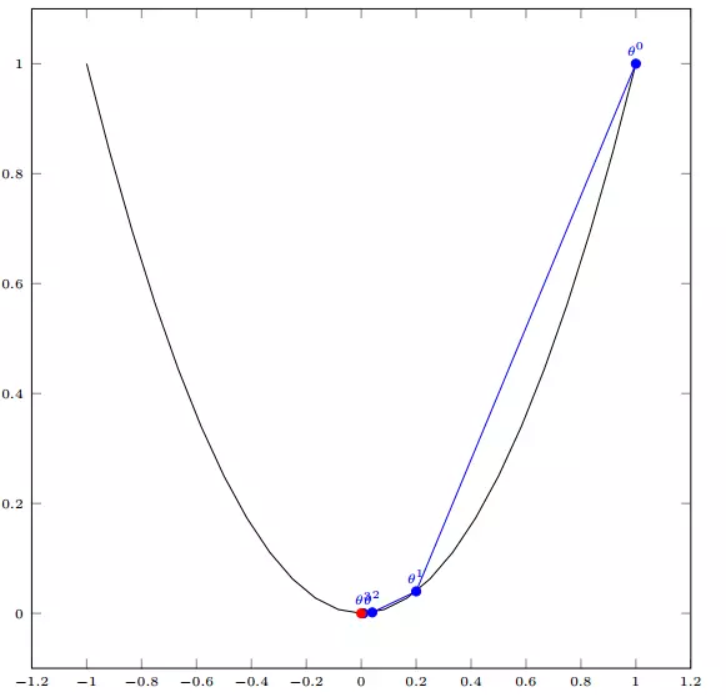3.2 多变量函数的梯度下降
我们假设有一个目标函数
$J(\Theta) = \theta_{1}^2 + \theta_{2}^2$
现在要通过梯度下降法计算这个函数的最小值。我们通过观察就能发现最小值其实就是 (0，0)点。但是接下来，我们会从梯度下降算法开始一步步计算到这个最小值！
我们假设初始的起点为：
$\Theta^0 = (1, 3)$
初始的学习率为：
$\alpha = 0.1$
函数的梯度为：
$\triangledown J(\Theta ) = \left < 2\theta_{1},2\theta_{2} \right >$
进行多次迭代：
$\Theta^0 = (1, 3)$
$\Theta^1 = \Theta^0 - \alpha\triangledown J(\Theta ) = (1,3) - 0.1*(2, 6)=(0.8, 2.4)$
$\Theta^2 = (0.8, 2.4) - 0.1*(1.6, 4.8)=(0.64, 1.92)$
$\Theta^3 =(0.5124, 1.536)$
$\Theta^4 =(0.4096, 1.228800000000001)$
$\vdots$
$\Theta^{10} =(0.1073741824000003, 0.32212254720000005)$
$\vdots$
$\Theta^{50} =(1.141798154164342e^{-05}, 3.42539442494306e^{-05})$
$\vdots$
$\Theta^{100} =(1.6296287810675902e^{-10}, 4.8888886343202771e^{-10})$
我们发现，已经基本靠近函数的最小值点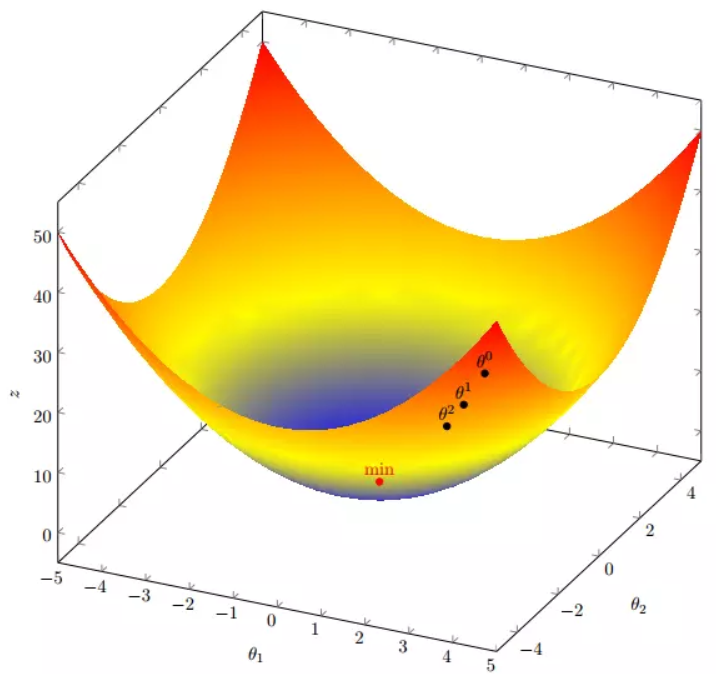4. 代码实现
4. 1 场景分析
下面我们将用python实现一个简单的梯度下降算法。场景是一个简单的线性回归的例子：假设现在我们有一系列的点，如下图所示：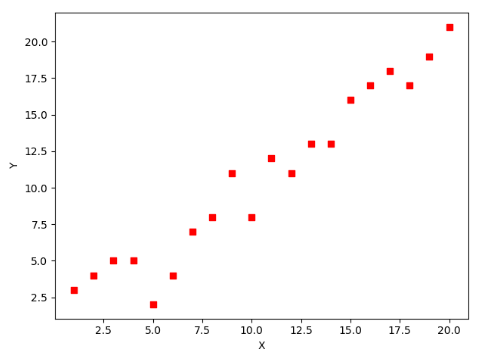我们将用梯度下降法来拟合出这条直线！
首先，我们需要定义一个代价函数，在此我们选用均方误差代价函数（也称平方误差代价函数）
$J(\Theta) = \frac{1}{2m}\sum_{i=1}^{m}(h_{\theta}(x^{(i)})-y^{(i)})^2$
此公式中

m是数据集中数据点的个数，也就是样本数
½是一个常量，这样是为了在求梯度的时候，二次方乘下来的2就和这里的½抵消了，自然就没有多余的常数系数，方便后续的计算，同时对结果不会有影响
y 是数据集中每个点的真实y坐标的值，也就是类标签
h 是我们的预测函数（假设函数），根据每一个输入x，根据Θ 计算得到预测的y值，即

$h_{\Theta}(x^{(i)}) = \Theta_{0} + \Theta_{1}x_{1}^{(i)}$
我们可以根据代价函数看到，代价函数中的变量有两个，所以是一个多变量的梯度下降问题，求解出代价函数的梯度，也就是分别对两个变量进行微分
$\triangledown J(\Theta ) = \left < \frac{\delta J}{\delta \Theta_{0}}, \frac{\delta J}{\delta \Theta_{1}} \right >$
$\frac{\delta J}{\delta \Theta_{0}} = \frac{1}{m}\sum_{i=1}^{m}(h_{\Theta}(x^{(i)})-y^{(i)})$
$\frac{\delta J}{\delta \Theta_{1}} = \frac{1}{m}\sum_{i=1}^{m}(h_{\Theta}(x^{(i)})-y^{(i)})x_{1}^{(i)}$
明确了代价函数和梯度，以及预测的函数形式。我们就可以开始编写代码了。但在这之前，需要说明一点，就是为了方便代码的编写，我们会将所有的公式都转换为矩阵的形式，python中计算矩阵是非常方便的，同时代码也会变得非常的简洁。
为了转换为矩阵的计算，我们观察到预测函数的形式
$h_{\Theta}(x^{(i)}) = \Theta_{0} + \Theta_{1}x^{(i)}$
我们有两个变量，为了对这个公式进行矩阵化，我们可以给每一个点x增加一维，这一维的值固定为1，这一维将会乘到Θ0上。这样就方便我们统一矩阵化的计算
$(x_{1}^{(i)},y^{(i)})\rightarrow (x_{0}^{(i)},x_{1}^{(i)},y^{(i)}) with x_{0}^{(i)} = 1 \forall _{i}$
然后我们将代价函数和梯度转化为矩阵向量相乘的形式
$J(\Theta) = \frac{1}{2m}(X\Theta - \vec{y})^{T}(X\Theta - \vec{y})$
$\triangledown J(\Theta) = \frac{1}{m}X^{T}(X\Theta - \vec{y}))$
4. 2 代码
首先，我们需要定义数据集和学习率
#!/usr/bin/env python3
# -*- coding: utf-8 -*-
# @Time    : 2019/1/21 21:06
# @Author  : Arrow and Bullet
# @Software: PyCharm
# @Blog    ：https://blog.csdn.net/qq_41800366

from numpy import *

# 数据集大小 即20个数据点
m = 20
# x的坐标以及对应的矩阵
X0 = ones((m, 1))  # 生成一个m行1列的向量，也就是x0，全是1
X1 = arange(1, m+1).reshape(m, 1)  # 生成一个m行1列的向量，也就是x1，从1到m
X = hstack((X0, X1))  # 按照列堆叠形成数组，其实就是样本数据
# 对应的y坐标
y = np.array([
3, 4, 5, 5, 2, 4, 7, 8, 11, 8, 12,
11, 13, 13, 16, 17, 18, 17, 19, 21
]).reshape(m, 1)
# 学习率
alpha = 0.01

接下来我们以矩阵向量的形式定义代价函数和代价函数的梯度
# 定义代价函数
def cost_function(theta, X, Y):
diff = dot(X, theta) - Y  # dot() 数组需要像矩阵那样相乘，就需要用到dot()
return (1/(2*m)) * dot(diff.transpose(), diff)

# 定义代价函数对应的梯度函数
diff = dot(X, theta) - Y
return (1/m) * dot(X.transpose(), diff)

最后就是算法的核心部分，梯度下降迭代计算
# 梯度下降迭代
theta = array([1, 1]).reshape(2, 1)
theta = theta - alpha * gradient
return theta

print('optimal:', optimal)
print('cost function:', cost_function(optimal, X, Y))

当梯度小于1e-5时，说明已经进入了比较平滑的状态，类似于山谷的状态，这时候再继续迭代效果也不大了，所以这个时候可以退出循环！
运行代码，计算得到的结果如下：
print('optimal:', optimal)  # 结果 [[0.51583286][0.96992163]]
print('cost function:', cost_function(optimal, X, Y))  # 1.014962406233101

通过matplotlib画出图像，
# 根据数据画出对应的图像
def plot(X, Y, theta):
import matplotlib.pyplot as plt
ax = plt.subplot(111)  # 这是我改的
ax.scatter(X, Y, s=30, c="red", marker="s")
plt.xlabel("X")
plt.ylabel("Y")
x = arange(0, 21, 0.2)  # x的范围
y = theta + theta*x
ax.plot(x, y)
plt.show()

plot(X1, Y, optimal)

所拟合出的直线如下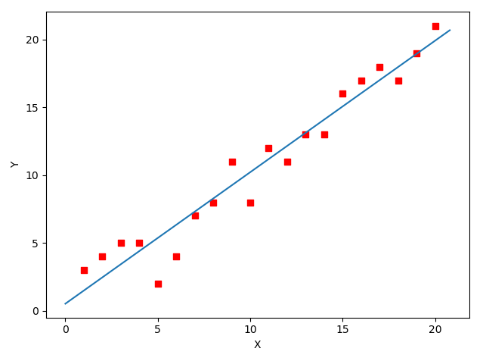全部代码如下，大家有兴趣的可以复制下来跑一下看一下结果：
#!/usr/bin/env python3
# -*- coding: utf-8 -*-
# @Time    : 2019/1/21 21:06
# @Author  : Arrow and Bullet
# @Software: PyCharm
# @Blog    ：https://blog.csdn.net/qq_41800366

from numpy import *

# 数据集大小 即20个数据点
m = 20
# x的坐标以及对应的矩阵
X0 = ones((m, 1))  # 生成一个m行1列的向量，也就是x0，全是1
X1 = arange(1, m+1).reshape(m, 1)  # 生成一个m行1列的向量，也就是x1，从1到m
X = hstack((X0, X1))  # 按照列堆叠形成数组，其实就是样本数据
# 对应的y坐标
Y = array([
3, 4, 5, 5, 2, 4, 7, 8, 11, 8, 12,
11, 13, 13, 16, 17, 18, 17, 19, 21
]).reshape(m, 1)
# 学习率
alpha = 0.01

# 定义代价函数
def cost_function(theta, X, Y):
diff = dot(X, theta) - Y  # dot() 数组需要像矩阵那样相乘，就需要用到dot()
return (1/(2*m)) * dot(diff.transpose(), diff)

# 定义代价函数对应的梯度函数
diff = dot(X, theta) - Y
return (1/m) * dot(X.transpose(), diff)

# 梯度下降迭代
theta = array([1, 1]).reshape(2, 1)
theta = theta - alpha * gradient
return theta

print('optimal:', optimal)
print('cost function:', cost_function(optimal, X, Y))

# 根据数据画出对应的图像
def plot(X, Y, theta):
import matplotlib.pyplot as plt
ax = plt.subplot(111)  # 这是我改的
ax.scatter(X, Y, s=30, c="red", marker="s")
plt.xlabel("X")
plt.ylabel("Y")
x = arange(0, 21, 0.2)  # x的范围
y = theta + theta*x
ax.plot(x, y)
plt.show()

plot(X1, Y, optimal)

5. 小结
至此，就基本介绍完了梯度下降法的基本思想和算法流程，并且用python实现了一个简单的梯度下降算法拟合直线的案例！
最后，我们回到文章开头所提出的场景假设:
这个下山的人实际上就代表了反向传播算法，下山的路径其实就代表着算法中一直在寻找的参数Θ，山上当前点的最陡峭的方向实际上就是代价函数在这一点的梯度方向，场景中观测最陡峭方向所用的工具就是微分 。在下一次观测之前的时间就是有我们算法中的学习率α所定义的。
可以看到场景假设和梯度下降算法很好的完成了对应！
本文部分内容来自一位前辈，非常感谢分享！谢谢！


展开全文梯度下降
• ## 一文看懂常用的梯度下降算法

万次阅读 多人点赞 2017-11-29 00:00:00


作者：叶    虎

编辑：祝鑫泉一概述

梯度下降算法（Gradient Descent Optimization）是神经网络模型训练最常用的优化算法。对于深度学习模型，基本都是采用梯度下降算法来进行优化训练的。梯度下降算法背后的原理：目标函数关于参数的梯度将是目标函数上升最快的方向。对于最小化优化问题，只需要将参数沿着梯度相反的方向前进一个步长，就可以实现目标函数的下降。这个步长又称为学习速率。参数更新公式如下：其中是参数的梯度，根据计算目标函数采用数据量的不同，梯度下降算法又可以分为批量梯度下降算法（Batch
Gradient Descent）。对于批量梯度下降算法，其是在整个训练集上计算的，如果数据集比较大，可能会面临内存不足问题，而且其收敛速度一般比较慢。随机梯度下降算法是另外一个极端，是针对训练集中的一个训练样本计算的，又称为在线学习，即得到了一个样本，就可以执行一次参数更新。所以其收敛速度会快一些，但是有可能出现目标函数值震荡现象，因为高频率的参数更新导致了高方差。小批量梯度下降算法是折中方案，选取训练集中一个小批量样本计算，这样可以保证训练过程更稳定，而且采用批量训练方法也可以利用矩阵计算的优势。这是目前最常用的梯度下降算法。

对于神经网络模型，借助于BP算法可以高效地计算梯度，从而实施梯度下降算法。但梯度下降算法一个老大难的问题是：不能保证全局收敛。如果这个问题解决了，深度学习的世界会和谐很多。梯度下降算法针对凸优化问题原则上是可以收敛到全局最优的，因为此时只有唯一的局部最优点。而实际上深度学习模型是一个复杂的非线性结构，一般属于非凸问题，这意味着存在很多局部最优点（鞍点），采用梯度下降算法可能会陷入局部最优，这应该是最头疼的问题。这点和进化算法如遗传算法很类似，都无法保证收敛到全局最优。因此，我们注定在这个问题上成为“高级调参师”。可以看到，梯度下降算法中一个重要的参数是学习速率，适当的学习速率很重要：学习速率过小时收敛速度慢，而过大时导致训练震荡，而且可能会发散。理想的梯度下降算法要满足两点：收敛速度要快；能全局收敛。为了这个理想，出现了很多经典梯度下降算法的变种，下面将分别介绍它们。

01

Momentum optimization
冲量梯度下降算法是BorisPolyak在1964年提出的，其基于这样一个物理事实：将一个小球从山顶滚下，其初始速率很慢，但在加速度作用下速率很快增加，并最终由于阻力的存在达到一个稳定速率。对于冲量梯度下降算法，其更新方程如下：可以看到，参数更新时不仅考虑当前梯度值，而且加上了一个积累项（冲量），但多了一个超参，一般取接近1的值如0.9。相比原始梯度下降算法，冲量梯度下降算法有助于加速收敛。当梯度与冲量方向一致时，冲量项会增加，而相反时，冲量项减少，因此冲量梯度下降算法可以减少训练的震荡过程。TensorFlow中提供了这一优化器：tf.train.MomentumOptimizer(learning_rate=learning_rate,momentum=0.9)。

02

NAG既然参数要沿着更新，不妨计算未来位置的梯度，然后合并两项作为最终的更新项，其具体效果如图1所示，可以看到一定的加速效果。在TensorFlow中，NAG优化器为：tf.train.MomentumOptimizer(learning_rate=learning_rate,momentum=0.9,
use_nesterov=True)图1 NAG效果图

0304

RMSprop可以认为仅仅对距离时间较近的梯度进行积累，其中一般取值0.9，其实这样就是一个指数衰减的均值项，减少了出现的爆炸情况，因此有助于避免学习速率很快下降的问题。同时Hinton也建议学习速率设置为0.001。RMSprop是属于一种比较好的优化算法了，在TensorFlow中当然有其身影：tf.train.RMSPropOptimizer(learning_rate=learning_rate,momentum=0.9,
decay=0.9, epsilon=1e-10)。

05可以看到前两项和Momentum和RMSprop是非常一致的，由于和的初始值一般设置为0，在训练初期其可能较小，第三和第四项主要是为了放大它们。最后一项是参数更新。其中超参数的建议值是。Adm是性能非常好的算法，在TensorFlow其实现如下：

二学习速率

前面也说过学习速率的问题，对于梯度下降算法，这应该是一个最重要的超参数。如果学习速率设置得非常大，那么训练可能不会收敛，就直接发散了；如果设置的比较小，虽然可以收敛，但是训练时间可能无法接受；如果设置的稍微高一些，训练速度会很快，但是当接近最优点会发生震荡，甚至无法稳定。不同学习速率的选择影响可能非常大，如图3所示。图3
不同学习速率的训练效果

理想的学习速率是：刚开始设置较大，有很快的收敛速度，然后慢慢衰减，保证稳定到达最优点。所以，前面的很多算法都是学习速率自适应的。除此之外，还可以手动实现这样一个自适应过程，如实现学习速率指数式衰减：在TensorFlow中，你可以这样实现：

initial_learning_rate = 0.1decay_steps = 10000decay_rate = 1/10global_step = tf.Variable(0, trainable=False)learning_rate = tf.train.exponential_decay(initial_learning_rate,                                                       global_step, decay_steps, decay_rate)# decayed_learning_rate = learning_rate *#                decay_rate ^ (global_step / decay_steps)optimizer = tf.train.MomentumOptimizer(learning_rate, momentum=0.9)training_op = optimizer.minimize(loss, global_step=global_step)

三总结

四
参考文献

Anoverview of gradient descent optimization algorithms:

Hands-OnMachine Learning with Scikit-Learn and TensorFlow, Aurélien Géron, 2017.

NAG:http://proceedings.mlr.press/v28/sutskever13.pdf.

RMSprop:http://www.cs.toronto.edu/~tijmen/csc321/slides/lecture_slides_lec6.pdf.

不同的算法的效果可视化：https://imgur.com/a/Hqolp.

欢迎大家加群在群中探讨
欢迎留言或赞赏。

推
荐
阅
读

客官，来嘛，谷歌小菜请你尝尝！

趣谈深度学习核心----激活函数

朴素贝叶斯实战篇之新浪新闻分类

Object
Detection R-CNN

史上最详细的XGBoost实战（上）

扫描个人微信号，
拉你进机器学习大牛群。
福利满满，名额已不多…80%的AI从业者已关注我们微信公众号展开全文• ## 梯度下降算法

千次阅读 2021-03-23 21:50:54
梯度下降算法 梯度下降算法的基本思想简介： 梯度下降算法又称最速下降法。梯度下降法的基本思想可以类比为一个下山的过程。 假设这样一个场景：一个人被困在山上，需要从山上下来(找到山的最低点，也就是山谷)。但...
梯度下降算法
梯度下降算法的基本思想简介：
梯度下降算法(欧式范数的最速下降法)。梯度下降法的基本思想可以类比为一个下山的过程。
假设这样一个场景：一个人被困在山上，需要从山上下来(找到山的最低点，也就是山谷)。但此时山上的浓雾很大，导致可视度很低；因此，下山的路径就无法确定，必须利用自己周围的信息一步一步地找到下山的路。这个时候，便可利用梯度下降算法来帮助自己下山。怎么做呢，首先以他当前的所处的位置为基准，寻找这个位置最陡峭的地方，然后朝着下降方向走一步，然后又继续以当前位置为基准，再找最陡峭的地方，再走直到最后到达最低处；同理上山也是如此，只是这时候就变成梯度上升算法了。
梯度下降算法思想的具体步骤：

选定初始点$x^1$和给定精度$\epsilon>0$,令$k=1$
若$\parallel\nabla f(x^k)\parallel<\epsilon$，停止梯度下降算法，$x^*=x^k$,否则$d^k=-\nabla f(x^k)$
在$x^k$处沿$d^k$方向作线性搜索，$x^{k+1}=x^k+\alpha d^k$,k=k+1,转步骤2.

例题：用最速下降法求解无约束问题
$min\lbrace f(x)=\cfrac{x_1^2}{2}+x_2^2\rbrace$
求解matlab代码附上：
本代码精度设置为$10^{-5}$
clc,clear
%%设定初始值
jingdu=1e-5%精度要求
x=[2 1];%初始点
%%梯度
fun_diff=[x(1),2*x(2)];
%%目标函数
fun=fun_ob(x)
%%梯度下降主循环
syms a%步长
while ((fun_diff(1)^2+fun_diff(2)^2)^0.5) > jingdu
%%梯度
fun_diff=[x(1),2*x(2)];
d=fun_diff*-1;
%一元线性搜索函数
k=fun_ob(x+a*d);
a_num=solve(diff(k));%求步长
x=x+a_num*d;
end
x,fun_ob(x)
function [a]=fun_ob(x)
a=(x(1)^2)/2+x(2)^2;
end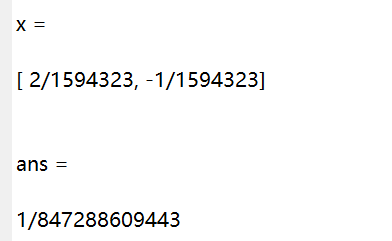其函数图像如下：并附上动态观察代码：
clc,clear
x1=-10:0.01:10
x2=-10:0.01:10
[x1,x2]=meshgrid(x1,x2)
x3=(x1.^2)/2+x2.^2;

%%设定初始值
jingdu=1e-5%精度要求
x=[10 10];%初始点
%%梯度
fun_diff=[x(1),2*x(2)];
%%目标函数
fun=fun_ob(x)
%%梯度下降主循环
syms a%步长
while ((fun_diff(1)^2+fun_diff(2)^2)^0.5) > jingdu
%%Draw
mesh(x1,x2,x3);
grid on
hold on
plot3(x(1),x(2),fun,'r*')
pause(0.1)

hold off
%%梯度
fun_diff=[x(1),2*x(2)];
d=fun_diff*-1;
%一元线性搜索函数
k=fun_ob(x+a*d);
a_num=solve(diff(k));%求步长
x=x+a_num*d;
fun=fun_ob(x)
end
x,fun_ob(x)
function [a]=fun_ob(x)
a=(x(1)^2)/2+x(2)^2;
end

附上求解结果对应位置：展开全文机器学习 matlab
• 文章目录线性回归学习目标2.5 梯度下降法介绍1 全梯度下降算法（FG）2 随机梯度下降算法（SG）3 小批量梯度下降算法（mini-bantch）4 随机平均梯度下降算法（SAG）5 算法比较6 梯度下降优化算法(拓展) 学习目标 ...
线性回归
文章目录线性回归学习目标2.5 梯度下降法介绍1 全梯度下降算法（FG）2 随机梯度下降算法（SG）3 小批量梯度下降算法（mini-bantch）4 随机平均梯度下降算法（SAG）5 算法比较6 梯度下降优化算法(拓展)
学习目标

掌握线性回归的实现过程
应用LinearRegression或SGDRegressor实现回归预测
知道回归算法的评估标准及其公式
知道过拟合与欠拟合的原因以及解决方法
知道岭回归的原理及与线性回归的不同之处
应用Ridge实现回归预测
应用joblib实现模型的保存与加载

2.5 梯度下降法介绍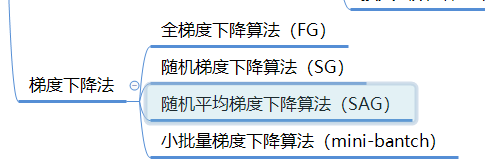上一节中给大家介绍了最基本的梯度下降法实现流程，常见的梯度下降算法有：

它们都是为了正确地调节权重向量，通过为每个权重计算一个梯度，从而更新权值，使目标函数尽可能最小化。其差别在于样本的使用方式不同。
1 全梯度下降算法（FG）
计算训练集所有样本误差，对其求和再取平均值作为目标函数。
权重向量沿其梯度相反的方向移动，从而使当前目标函数减少得最多。
因为在执行每次更新时，我们需要在整个数据集上计算所有的梯度，所以批梯度下降法的速度会很慢，同时，批梯度下降法无法处理超出内存容量限制的数据集。
批梯度下降法同样也不能在线更新模型，即在运行的过程中，不能增加新的样本。
其是在整个训练数据集上计算损失函数关于参数θ的梯度：2 随机梯度下降算法（SG）
由于FG每迭代更新一次权重都需要计算所有样本误差，而实际问题中经常有上亿的训练样本，故效率偏低，且容易陷入局部最优解，因此提出了随机梯度下降算法。
其每轮计算的目标函数不再是全体样本误差，而仅是单个样本误差，即每次只代入计算一个样本目标函数的梯度来更新权重，再取下一个样本重复此过程，直到损失函数值停止下降或损失函数值小于某个可以容忍的阈值。
此过程简单，高效，通常可以较好地避免更新迭代收敛到局部最优解。其迭代形式为每次只使用一个样本迭代，若遇上噪声则容易陷入局部最优解。

其中，x(i)表示一条训练样本的特征值，y(i)表示一条训练样本的标签值

但是由于，SG每次只使用一个样本迭代，若遇上噪声则容易陷入局部最优解。
3 小批量梯度下降算法（mini-bantch）
小批量梯度下降算法是FG和SG的折中方案,在一定程度上兼顾了以上两种方法的优点。
每次从训练样本集上随机抽取一个小样本集，在抽出来的小样本集上采用FG迭代更新权重。
被抽出的小样本集所含样本点的个数称为batch_size，通常设置为2的幂次方，更有利于GPU加速处理。
特别的，若batch_size=1，则变成了SG；若batch_size=n，则变成了FG.其迭代形式为4 随机平均梯度下降算法（SAG）
在SG方法中，虽然避开了运算成本大的问题，但对于大数据训练而言，SG效果常不尽如人意，因为每一轮梯度更新都完全与上一轮的数据和梯度无关。
随机平均梯度算法克服了这个问题，在内存中为每一个样本都维护一个旧的梯度，随机选择第i个样本来更新此样本的梯度，其他样本的梯度保持不变，然后求得所有梯度的平均值，进而更新了参数。
如此，每一轮更新仅需计算一个样本的梯度，计算成本等同于SG，但收敛速度快得多。
5 算法比较
以下6幅图反映了模型优化过程中四种梯度算法的性能差异。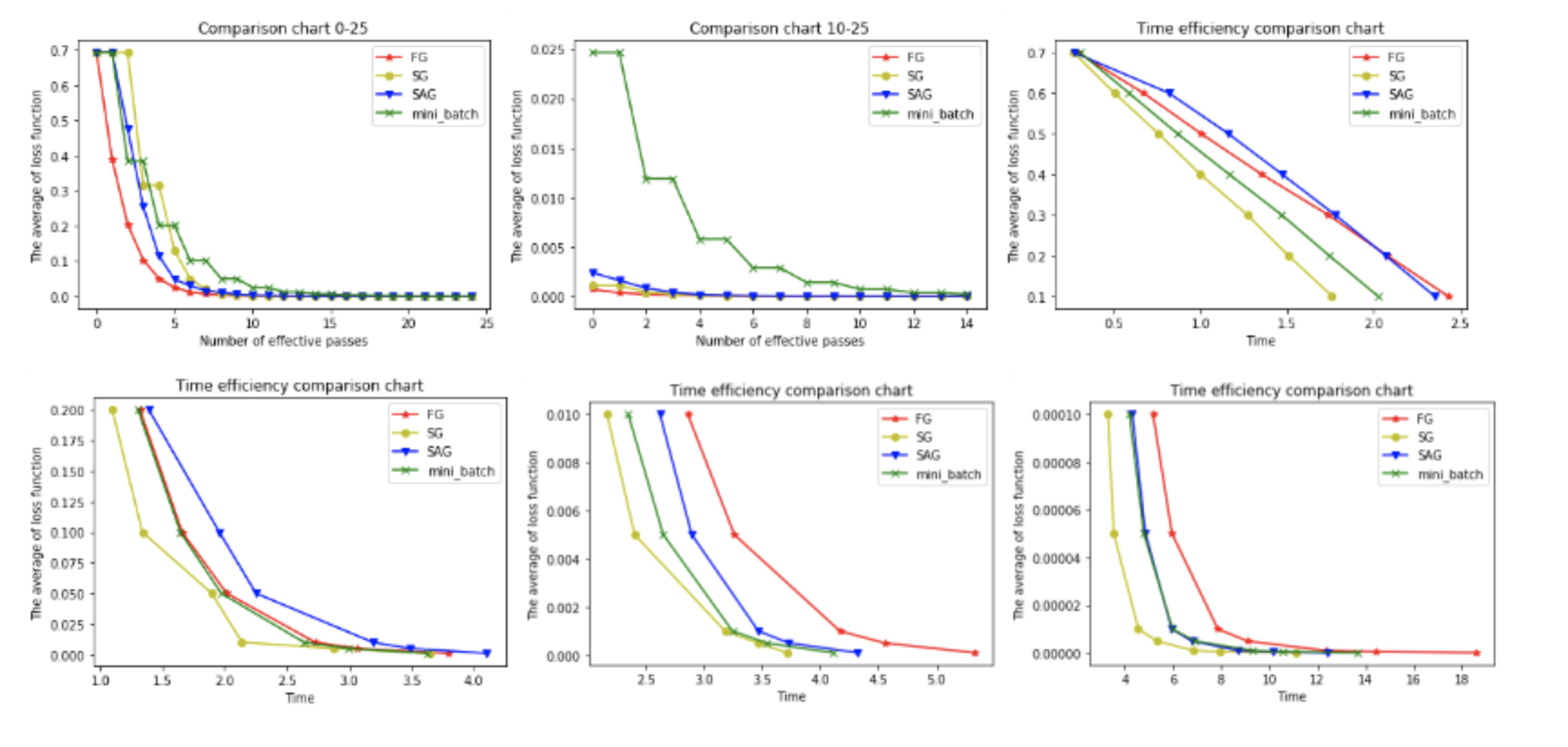在图1和图2中，横坐标代表有效迭代次数，纵坐标代表平均损失函数值。图1反映了前25次有效迭代过程中平均损失函数值的变化情况，为了便于观察，图2放大了第10次到25次的迭代情况。
从图1中可以看到，四种梯度算法下，平均损失函数值随迭代次数的增加而减少。FG的迭代效率始终领先，能在较少的迭代次数下取得较低的平均损失函数值。FG与SAG的图像较平滑，这是因为这两种算法在进行梯度更新时都结合了之前的梯度；SG与mini-batch的图像曲折明显，这是因为这两种算法在每轮更新梯度时都随机抽取一个或若干样本进行计算，并没有考虑到之前的梯度。
从图2中可以看到**虽然四条折现的纵坐标虽然都趋近于0，但SG和FG较早，mini-batch最晚。**这说明如果想使用mini-batch获得最优参数，必须对其进行较其他三种梯度算法更多频次的迭代。
在图3，4，5，6中，横坐标表示时间，纵坐标表示平均损失函数值。
从图3中可以看出使用四种算法将平均损失函数值从0.7降到0.1最多只需要2.5s，由于本文程序在初始化梯度时将梯度设为了零，故前期的优化效果格外明显。其中SG在前期的表现最好，仅1.75s便将损失函值降到了0.1，虽然SG无法像FG那样达到线性收敛，但在处理大规模机器学习问题时，为了节约时间成本和存储成本，可在训练的一开始先使用SG，后期考虑到收敛性和精度可改用其他算法。
从图4，5，6可以看出，随着平均损失函数值的不断减小，SG的性能逐渐反超FG，FG的优化效率最慢，即达到相同平均损失函数值时FG所需要的时间最久。
综合分析六幅图我们得出以下结论：
（1**）FG方法由于它每轮更新都要使用全体数据集，故花费的时间成本最多，内存存储最大。**
（2）SAG在训练初期表现不佳，优化速度较慢。这是因为我们常将初始梯度设为0，而SAG每轮梯度更新都结合了上一轮梯度值。
（3）综合考虑迭代次数和运行时间，SG表现性能都很好，能在训练初期快速摆脱初始梯度值，快速将平均损失函数降到很低。但要注意，在使用SG方法时要慎重选择步长，否则容易错过最优解。
（4）mini-batch结合了SG的“胆大”和FG的“心细”，从6幅图像来看，它的表现也正好居于SG和FG二者之间。在目前的机器学习领域，mini-batch是使用最多的梯度下降算法，正是因为它避开了FG运算效率低成本大和SG收敛效果不稳定的缺点。
6 梯度下降优化算法(拓展)
以下这些算法主要用于深度学习优化

动量法

其实动量法(SGD with monentum)就是SAG的姐妹版
SAG是对过去K次的梯度求平均值
SGD with monentum 是对过去所有的梯度求加权平均

Nesterov加速梯度下降法

类似于一个智能球，在重新遇到斜率上升时候，能够知道减速

让学习率使用参数
对于出现次数较少的特征，我们对其采用更大的学习率，对于出现次数较多的特征，我们对其采用较小的学习率。

RMSProp

其结合了梯度平方的指数移动平均数来调节学习率的变化。
能够在不稳定（Non-Stationary）的目标函数情况下进行很好地收敛。

是一种自适应的学习率算法


展开全文python 神经网络 机器学习 人工智能
• 文章目录2.5 梯度下降法介绍学习目标1 全梯度下降算法（FG）2 随机梯度下降算法（SG）3 小批量梯度下降算法（mini-batch）4 随机平均梯度下降算法（SAG）5 小结 2.5 梯度下降法介绍 学习目标 知道全梯度下降算法的...机器学习
• 梯度下降算法的正确步骤Title: What is the Gradient Descent Algorithm and its working. 标题:什么是梯度下降算法及其工作原理。 Gradient descent is a type of machine learning algorithm that helps us in ...深度学习 python 机器学习 人工智能
• 梯度下降法、随机梯度下降算法、批量梯度下降 梯度下降：梯度下降就是我上面的推导，要留意，在梯度下降中，对于θ的更新，所有的样本都有贡献，也就是参与调整θ 其计算得到的是一个标准梯度。因而理论上来说一次...机器学习
• 批量梯度下降算法和随机梯度下降算法的代码实现 随机梯度下降和批量梯度下降都是梯度下降方法的一种，都是通过求偏导的方式求参数的最优解。批量梯度下降算法：，。是通过对每一个样本求偏导，然后挨个更新。...
• 梯度下降算法 梯度下降，依照所给数据，判断函数，随机给一个初值w，之后通过不断更改，一步步接近原函数的方法。更改的过程也就是根据梯度不断修改w的过程。 以简单的一元函数为例 原始数据为 x_data = [1.0,...python
• 一、一维梯度下降 算法思想： 我们要找到一个函数的谷底，可以通过不断求导，不断逼近，找到...和一维梯度下降算法思想类似，只是导数由原来的一维变成现在的多维，算法思想本质没有变化，在计算导数的过程发生了...
• 转载自：https://www.cnblogs.com/HongjianChen/p/8718988.html梯度下降算法：w=w-α∇wf(w)梯度上升算法：w=w+α∇wf(w)梯度上升算法用来求函数的最大值， 梯度下降算法用来求函数的最小值。方向导数当讨论函数沿...机器学习
• 在学习线性回归的时候很多课程都会讲到用梯度下降法求解参数，对于梯度下降算法怎么求出这个解讲的较少，自己实现一遍算法比较有助于理解算法，也能注意到比较细节的东西。具体的数学推导可以参照这一篇博客...python实现...